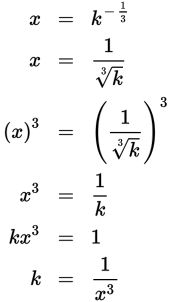# SAT Math Multiple Choice Question 249: Answer and Explanation

### Test Information

Question: 249

9. If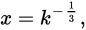where x > 0 and k > 0, which of the following equations gives k in terms of x?

• A.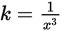• B.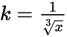• C.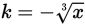• D. k = -x3

Explanation:

A

Difficulty: Medium

Category: Passport to Advanced Math / Exponents

Strategic Advice: When you write an equation in terms of a specific variable, you are simply solving the equation for that variable. In this question, you'll need to relate fractional exponents to radicals and understand how to use negative exponents. Be careful-you're not just rewriting the equation, you're solving it for k.

Getting to the Answer: Raising a quantity to the one-third power is the same as taking its cube root. Applying a negative exponent to a quantity is the same as writing its reciprocal. Rewrite the equation using these properties and then solve for k using inverse operations. Note that the inverse of taking a cube root of a quantity is cubing the quantity.